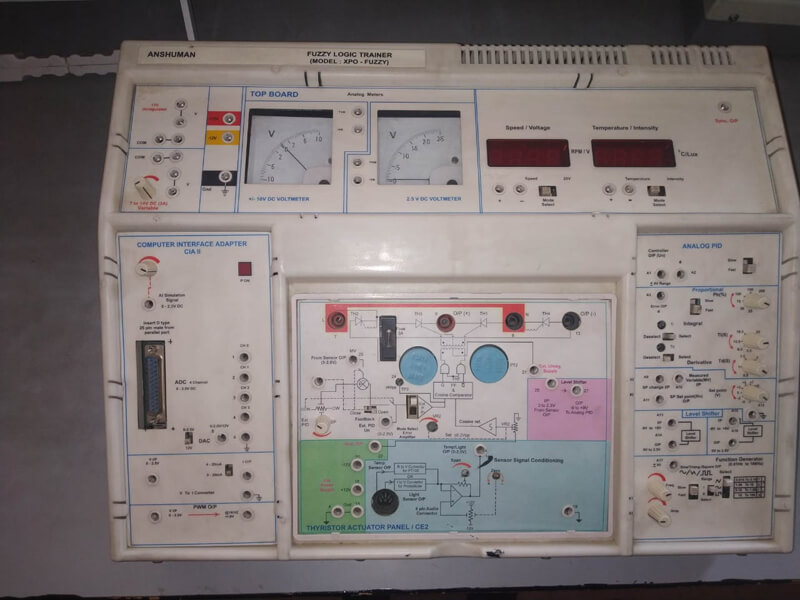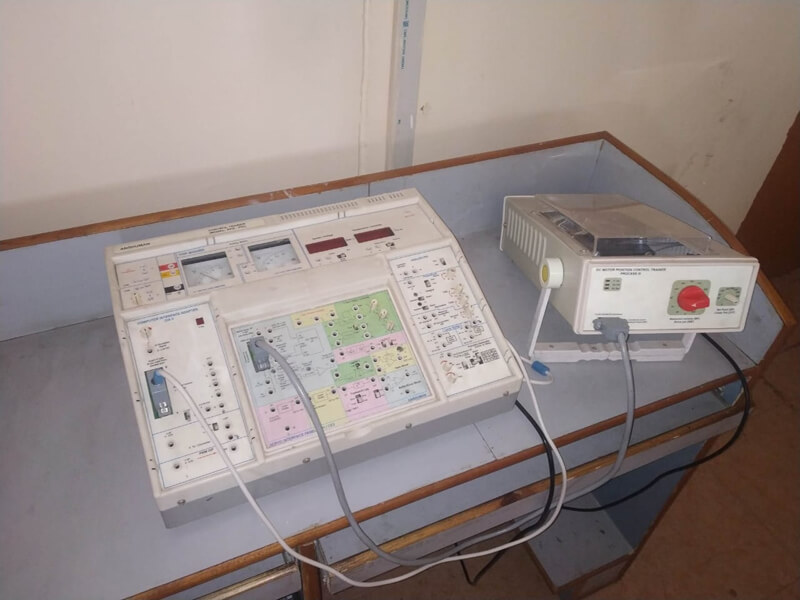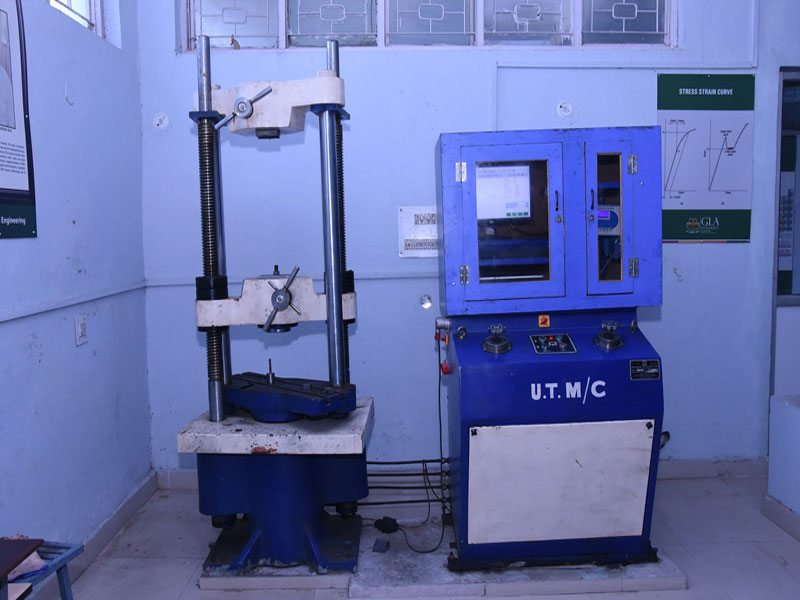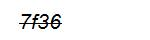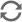### Basic Electrical and Electronics Engineering Lab

The Electrical Engineering laboratory is one of the important lab of the department as it helps the students of all engineering disciplines to perform the experiments based on basic concepts of electrical science. It is well-equipped with several experimental setups packages for real time experiments.

In this lab, the students of all engineering streams are trained on basic concepts of electrical engineering., such as AC to DC conversion, measurement, transformer, verification of basic laws and theorems, use of CRO, DSO, etc. This lab has maximum utilization, handling approximately six hundred fifty students per semester.

Major Equipments:

• Single-phase Transformer
• Single-phase Auto transformer
• Wattmeter, Ammeter, Voltmeter with various rating
• Single-phase Induction motor
• Function generator, CRO, DSO
• DC and AC supply system with various rating
• RLC and logic gate kit
• Analog and digital energy meter testing panel

Simulation Software:

• MATLAB
• Pspice